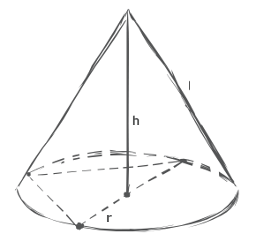# Right Circular Cone Calculator

## Definition

Cone -  a flat based three dimensional figure that consists of a flat base and a right angled axis that passes from the base to the apex. The base can be circular or polygonal, in which case the figure is called a pyramid.

Equation form:

 l = √(h²+r²) h = √(l²-r²) Surface Area (SA) = π * r * l
 Volume (V) = π * r² * h 3
Volume (VC) of circumscribed sphere about cone with radius r, height h, lateral height l:
 VC = π * l⁶ = π * l⁶ 6 * h³ 6 * (l²-r²) * √(l²-r²)### About Right Circular Cone Calculator tool.

Remember this tool should be used only to calculate area, perimeter or volume of a figure. The results we provide are accurate, but rounded to the 12th decimal place. It accepts scientific notation and converts immediately.
Tools categories
Popular tools
New tools